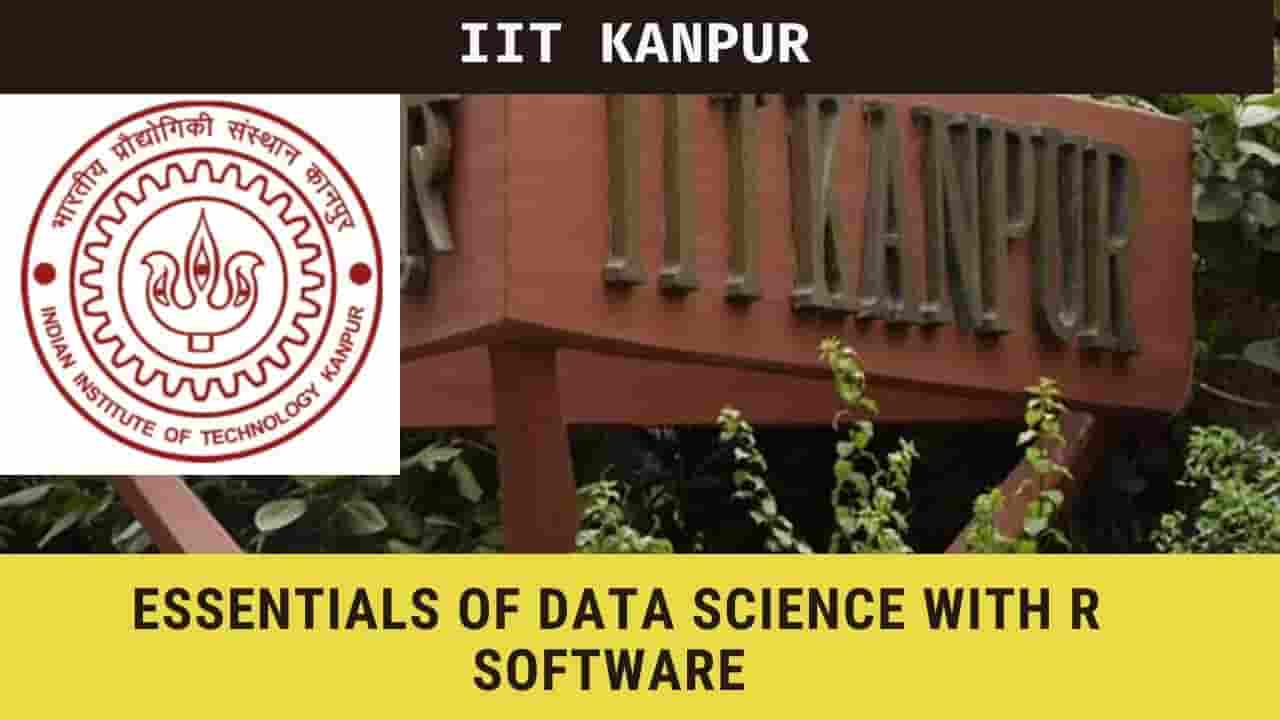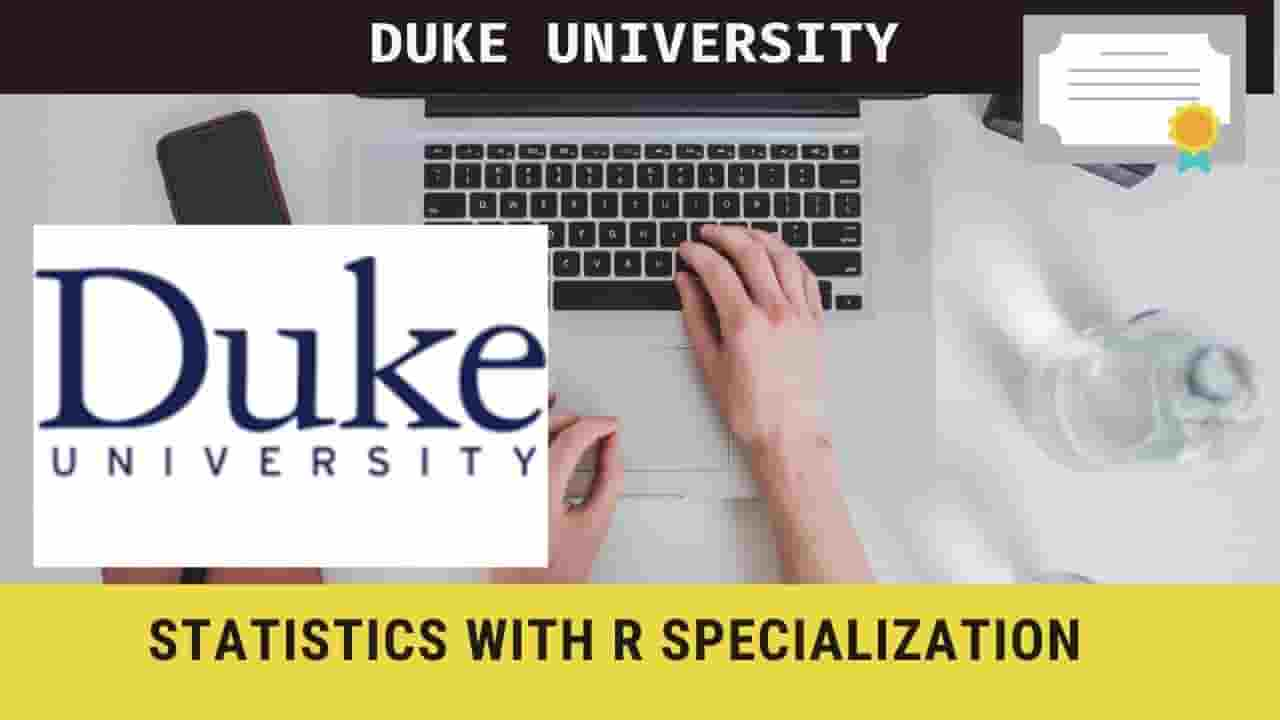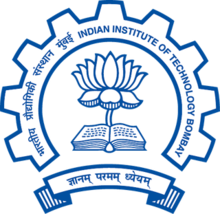# IIT Kanpur FREE course Essentials of Data Science With R Software - 1Essentials of Data Science With R Software - 1: Probability and Statistical Inference

IIT Kanpur

## Overview

IIT Kanpur has launched the online course on the Essentials of Data Science With R Software - 1: Probability and Statistical Inference. This is a 12-week course and will start from the 18th Jan 2021.

## Topics

The following topics will be covered in this online course,

• Introduction to data science, basic calculations with R Software and probability theory
• Probability theory and random variables
• Random variables and Discrete probability distributions
• Continuous probability distributions
• Sampling distributions and Functions of random variables
• The convergence of random variables, Central limit theorems, and Law of large numbers
•  Statistical inference and point estimation
• Methods of point estimation of parameters
• Point and confidence interval estimation
• Confidence interval estimation and test of hypothesis
• Test of hypothesis
• Test of hypothesis for attributes and other tests

## Eligibility

The following candidates  can apply in this course on Data science by the IIT Kanpur,

UG students of Science and Engineering. Students of humanities with a basic mathematical and statistical background can also do it. Working professionals in analytics can also do it.

## PREREQUISITES

“Introduction to R Course” is preferred. A mathematics background up to class 12 is needed. Some minor statistics background is desirable.

By Prof. Shalabh

## Last date

25 Jan 2021

#### For more (source) IIT Kanpur FREE course Essentials of Data Science With R Software - 1

##### Create a FREE webpage for your event.